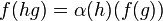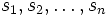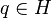# Induced representation

## Definition

### In abstract terms

Suppose$G$ is a group,$H$ is a subgroup of$G$, and$\alpha:H \to GL(V)$ is a linear representation of$H$ on a vector space$V$ over a field$K$. The induced representation of$G$ is a linear representation of$G$ on a new (bigger) vector space$W$ over the same field$K$, defined as follows.

1.$W$ is defined as the$K$-vector space of functions (under pointwise addition and scalar multiplication)$f:G \to V$ satisfying$\! f(hg) = \alpha(h)(f(g))$ for all$h \in H$ and$g \in G$. Note here that$f(g) \in V$ while$\alpha(h) \in GL(V)$ is a linear transformation of$V$, so it makes sense to apply$\alpha(h)$ to$f(g)$. The dimension of$W$ is the product of the dimension of$V$ and the index$[G:H]$ of$H$ in$G$.
2. The action of$G$ on$W$ is defined by the following map$\beta:G \to GL(W)$. For$g \in G$,$\beta(g)$ is the linear transformation that sends$f \in W$ to the following function$f_0$:$f_0(k) := f(kg)$ for$k \in G$. Note that the$g$ gets multiplied on the right in order to make this a left action, because the multiplication is happening on the inside rather than the outside.

### In matrix terms

Using the same notation as the previous definition, this more concrete description works when the index$[G:H]$ as well as the degree of$\alpha$ are both finite. Suppose$[G:H] = n$ and$\alpha$ has degree$m$, with$V$ identified with$K^m$ via a basis, so that$\alpha$ is now a map from$H$ to$GL(m,K)$. Then, we do the following:

• Choose a left transversal of$H$ in$G$, i.e., a set$S$ that intersects every left coset of$H$ in$G$ at exactly one point. Choose a bijection between this left transversal and$\{ 1,2,\dots,n\}$, and label the coset representatives$s_1, s_2, \dots, s_n$.
• If$\beta$ is the induced representation, we define$\beta(g)$, for any$g \in G$, as the following$mn \times mn$ matrix. We first begin by viewing it as a$n \times n$ block matrix with each block a$m \times m$ matrix. Define$q = s_i^{-1}gs_j$. For$i,j \in \{ 1,2,\dots,n\}$, the$ij^{th}$ block is defined as$\alpha(q)$ if$q \in H$ and$0$ otherwise. Note that the matrix is a block monomial matrix in the sense that, as a block matrix, every row has exactly one nonzero block and every column has exactly one nonzero block.

## Facts

### Iteration

• Induction of representations is transitive: If$A \le B \le C$ are groups then$\operatorname{Ind}_B^C \circ \operatorname{Ind}_A^B = \operatorname{Ind}_A^C$.